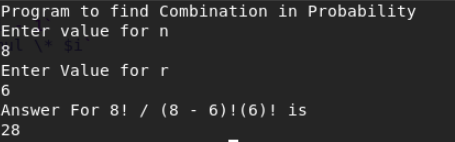# Program to Calculate Combination [nCr] in Bash: Shell Scripting

The following shell scripting program is written in bash to calculate combination formula which is n! /(n-r)!r!.

##### Code:
```#!/bin/bash
clear
echo "Program to find Combination in Probability"
function fact {
mul=1
i=\$1
while [[ \$i -gt 1 ]]
do
sub=`expr \$i - 1`
mul=`expr \$mul \* \$i`
i=\$sub
done
echo \$mul
}

echo "Enter value for n"
a=`fact \$n`
echo "Enter Value for r"
b=`fact \$r`
c=`expr "\$n" - "\$r"`
d=`fact \$c`
t=`expr "\$b" \* "\$d"`
ans=`expr "\$a" / "\$t"`

echo "Answer For \$n! / (\$n - \$r)!(\$r)! is"
echo "\$ans"
```

OUTPUT:This site uses Akismet to reduce spam. Learn how your comment data is processed.